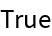#Function Repository Resource:

# Derangements

Give all the ways the numbers 1, 2, …, n can be scrambled completely

Contributed by: Wolfram Staff
 ResourceFunction["Derangements"][n] gives all lists that are rearrangements of {1,2,…,n} such that no element appears in its original position.

## Examples

### Basic Examples

In the following two lists, no element appears in its original place:

 In:=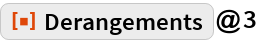Out=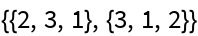Here is the next case, condensed:

 In:=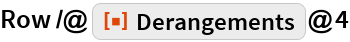Out=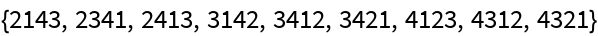### Scope

The number of lists grows with n!/:

 In:=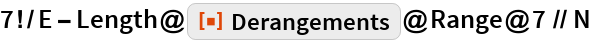Out=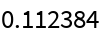In fact, the number of derangements is given by the built-in subfactorial function:

 In:=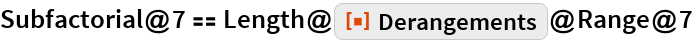Out=